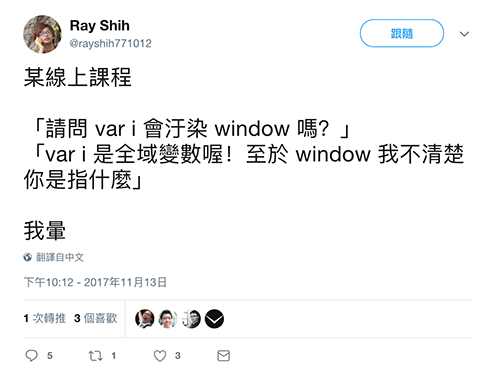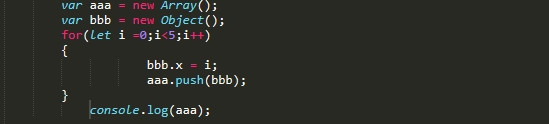#2018 iT 邦幫忙鐵人賽DAY 10
13
Modern Web

## 函式

「函式」指的是將一或多段程式指令包裝起來，可以重複使用，也方便維護。

• 函式的名稱 (也可能沒有名稱，稍後會提到)
• 在括號 `( )` 中的部分，稱為「參數 (arguments) 」，參數與參數之間會用逗號 `,` 隔開
• 在大括號 `{ }` 內的部分，內含需要重複執行的內容，是函式功能的主要區塊。

``````function square(number) {
return number * number;
}

square(2);        // 4
square(3);        // 9
square(4);        // 16
``````

## 定義函式的方式

• 函式宣告（Function Declaration）
• 函式運算式（Function Expressions）
• 透過 `new Function` 關鍵字建立函式

### 函式宣告（Function Declaration）

「函式宣告」應該是屬於最常見的用法：

``````function 名稱([參數]) {
// 做某事
}
``````

``````function square(number) {
return number * number;
}
``````

### 函式運算式（Function Expressions）

``````var square = function (number) {
return number * number;
};
``````

#### 沒有名字的函式？

``````var square = function (number) {
return number * number;
};
``````

``````var square = function func(number) {
return number * number;
};
``````

``````var square = function func(number) {
console.log( typeof func );   // "function"
return number * number;
};

console.log( typeof func );     // undefined
``````

``````var square = function func(number) {
console.log( typeof square );   // "function"
return number * number;
};
``````

### 透過 `new Function` 關鍵字建立函式

``````// 透過 new 來建立 Function "物件"
var square = new Function('number', 'return number * number');
``````

## 變數的有效範圍 (Scope)

``````var x = 1;

var doSomeThing = function(y) {
var x = 100;
return x + y;
};

console.log( doSomeThing(50) );   // ?
console.log( x );                 // ?
``````

.
.
.

「切分變數有效範圍的最小單位是 "function" 」
「切分變數有效範圍的最小單位是 "function" 」
「切分變數有效範圍的最小單位是 "function" 」

``````var x = 1;
var doSomeThing = function(y) {
// 內部找不到 x 就會到外面找，直到全域變數為止。
// 都沒有就會報錯：ReferenceError: x is not defined
return x + y;
};

console.log( doSomeThing(50) );   // 51
``````

### 沒有 `var` 宣告的變數很危險！

「沒有 `var` 宣告的變數很危險」什麼意思？

``````var x = 1;

var doSomeThing = function(y) {
x = 100;
return x + y;
};

console.log( doSomeThing(50) );   // ?
console.log( x );                 // ?
``````

.
.
.``````var doSomeThing = function(y) {
x = 100;
return x + y;
};
``````

### 提升 (Hoisting)

``````var x = 1;

var doSomeThing = function(y) {
console.log(x);   // 會出現什麼？

var x = 100;
return x + y;
};

console.log( doSomeThing(50) );   // 150
console.log( x );                 // 1
``````

.
.
.

.
.
.``````var x = 1;

var doSomeThing = function(y) {
var x;
console.log(x);   // 會出現什麼？

x = 100;
return x + y;
};

console.log( doSomeThing(50) );   // 150
console.log( x );                 // 1
``````

(還記得前面介紹變數時講過的嗎？ 只要變數有被宣告，使用時就不會有錯誤，否則會出現 `ReferenceError` 的錯誤。)

``````var doSomeThing = function(y) {
var x;
console.log(x);   // undefined

x = 100;
return x + y;
};
``````

``````square(2);    // 4

function square(number) {
return number * number;
}
``````

``````square(2);    // TypeError: square is not a function

var square = function (number) {
return number * number;
};
``````

## 全域變數與區域變數

#### 什麼叫做全域物件的「屬性」呢？

``````var a = 10;
``````

``````var a = 10;
console.log( window.a );    // ?
``````

• 變數有效範圍 (scope) 的最小切分單位是 `function` (ES6 的 `let``const` 例外)
• 即使是寫在函式內，沒有 `var` 的變數會變成「全域變數」
• 全域變數指的是全域物件 (頂層物件) 的「屬性」• [註1] 函式呼叫：除了單純的 `函式()` 之外，還有 `.call()``.apply()`，在後續的篇章介紹 `this` 時會提到這些。

• [註2] ES6 之後有 `let``const` 分別定義「變數」與「常數」。 與 `var` 不同的是，它們的 scope 是透過大括號 `{ }` 來切分的。

• [註3] 提升：提升看起來是將變數和函數的宣告移動到程式區塊頂端，但實際上是變數和函數的宣告會在編譯階段中先被放入記憶體，實際在程式碼中位置還是一樣，往上移動的說法是為了幫助理解。

• 到底 HTML、CSS 與 JavaScript 是什麼樣的關係？
• BOM 與 DOM 又是什麼東西？ 可以吃嗎
• JavaScript 怎麼操作我們的網頁？
• 網頁事件又是怎麼一回事？### 5 則留言

0

Kuro Hsu iT邦新手 4 級 ‧ 2017-12-14 09:41:48 檢舉0Kuro Hsu iT邦新手 4 級 ‧ 2019-06-02 22:33:05 檢舉

because object is call by reference.

``````var a = new Array();
var b = new Object();

for( let i = 0; i < 5; i++ ){
b.x = i;
console.log( b );
}
``````

and it will print

``````{ x: 0 }
{ x: 1 }
{ x: 2 }
{ x: 3 }
{ x: 4 }
``````

Kuro Hsu iT邦新手 4 級 ‧ 2019-06-02 22:59:16 檢舉

IIFE 那篇與你的問題是兩件事。

``````var a = new Array();

for( let i = 0; i < 5; i++ ){
let b = new Object();
b.x = i;
a.push( b );
}

console.log(a);
``````

0

Kuro 大大您好

``````var x = 1;

var doSomeThing = function(y) {
x = 100;
return x + y;
};

console.log( x ); //1
console.log( doSomeThing(50) );   //150
``````

Kuro Hsu iT邦新手 4 級 ‧ 2019-07-26 11:25:36 檢舉

``````var x = 1;

var doSomeThing = function(y) {
x = 100;
return x + y;
};

console.log( x ); // 1
console.log( doSomeThing(50) );   // 150

console.log( x );  // 1 or 100 ?
``````

`doSomeThing` 裡頭的 `x = 100;` 改成 `var x = 100;` 後，又會有什麼不同 :)

chunwen iT邦新手 5 級 ‧ 2019-07-26 14:05:19 檢舉

0

Kuro 老師 你好：

``````// 若是在全域的狀況下

var a = 10;   //使用 var 宣告
b = 10;    // 不使用 var

// 是不是都是一樣的宣告方式？因為

console.log(a);   // 10
console.log(b);   // 10
console.log(window.a);   // 10
console.log(window.b);   // 10

``````

Kuro Hsu iT邦新手 4 級 ‧ 2020-04-06 18:10:26 檢舉

``````a = 1000;

(function (){
var a = 10;

(function (){
a = 20;
console.log(a);
})()

console.log(a);
})()

console.log(a);
``````

Alec iT邦新手 5 級 ‧ 2020-04-06 19:14:57 檢舉

Kuro Hsu iT邦新手 4 級 ‧ 2020-04-07 10:17:13 檢舉

Alec iT邦新手 5 級 ‧ 2020-04-07 14:14:51 檢舉0

Kuro Hsu iT邦新手 4 級 ‧ 2020-08-05 10:43:38 檢舉